# Isometric Sketch

Isometric Sketch or isometric drawing is a pictorial representation of an object in which all three dimensions are drawn at full scale. It looks like an isometric projection. In this case, all the lines parallel to its major axes are measurable. In this article, you can understand what is an isometric sketch, the rules for an isometric sketch and examples.

## What is Isometric Sketch?

Three-dimensional objects can be represented on a two-dimensional plane easily if appropriately drawn. Oblique sketching is a method of representing 3-D objects on a 2-D plane. Although oblique sketches are capable enough to project the correct image of the object, the actual measurement of the object may vary as compared to the image projected on a two-dimensional plane. To overcome this limitation, the isometric sketch is used, which portrays the exact measurement of the object along with the projection of its image on two dimensions. Important points of an isometric drawing are:

1. The meaning of isometric is having equal measurements or dimensions.
2. In an isometric sketch, the image of the object is drawn in such a manner that the original dimension of the object is intact irrespective of its position.

Isometric sketching allows us to draw an object in the three-dimensions. This kind of sketching is often used by illustrators, engineers that specialize in technical drawings.

An isometric sketch is one of the types of axonometric drawings. The other two types are dimetric and oblique sketches.

## Rules for Isometric Sketching

Some of the rules for isometric sketching are as follows:

• We know that an isometric object can be drawn using vertical lines and horizontal lines. In the isometric drawing, the vertical lines will stay vertically, whereas the horizontal lines are drawn at an angle of 30-degree  to the horizontal plane.
• The angle between all the three axes of the coordinate plane must be equal to 120 degrees. If you follow rule 1, then rule 2 can be automatically achieved.
• The isometric projection displays the three faces of an object, and they all are uniformly foreshortened.

## Uses of Isometric Sketch

• Any objects that can be drawn using the isometric projection give a clear perception of the object.
• From the isometric sketching, one can take actual measurements of the object.
• Isometric projections should be extensively used in the situation when if you have a product idea in mind, and you want to show it to your Boss or an Investor. It would help if you showed your plan by drawing it using isometric sketching.
• It is broadly used by various professionals such as Game Designers, Architects, Interior designers, Infographics and so on.

## Isometric Sketch of Cube

An isometric sketch of a cube represents three faces that resemble three equilateral parallelograms. The parallel edges of the cube appear as parallel lines. The horizontal edges of the cube are drawn at an angle from the horizontal axes and vertical edges are parallel to the principal axes. All the vertical lines are drawn in proportion.

## Isometric Sketch Example

An example to draw the isometric sketching is given below. Follow the steps given to draw an isometric sketch of an 8 × 3 × 3 cuboid. The same steps can be used to draw an isometric sketch of a cube also.

Step 1. To draw an isometric sketch of a cuboid with dimension 8 × 3 × 3, take an isometric dot paper as shown below: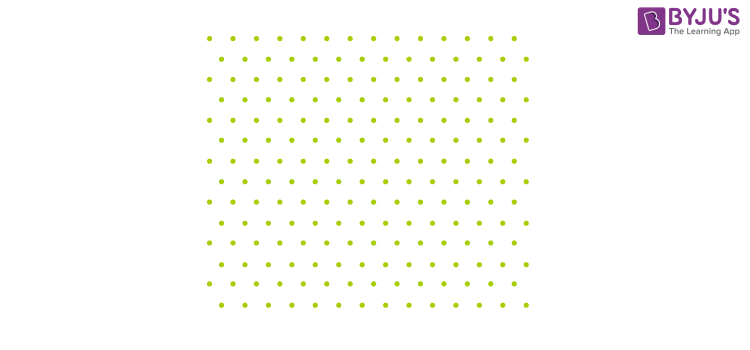Step 2. To draw the front face, join 8 dots to form the length of the cuboid and 3 adjacent dots to form its breadth as shown: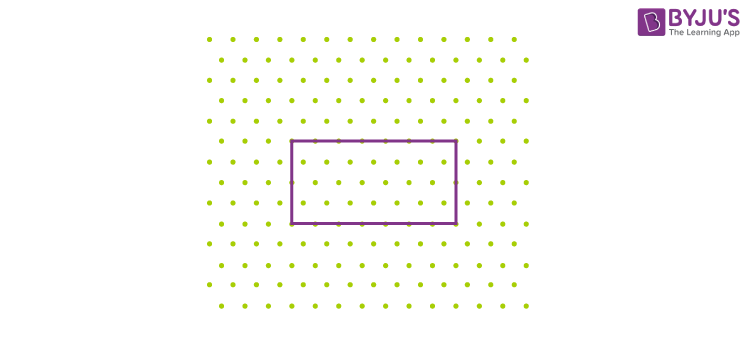Step 3. From the corners of the rectangle drawn above, draw 4 parallel line segments as shown below: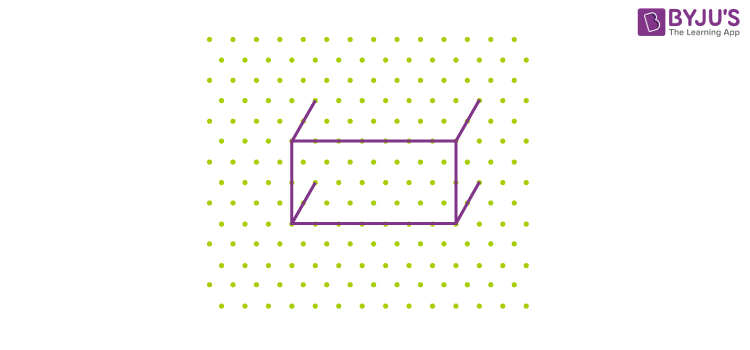Step 4. Join the corners of the image together as shown below: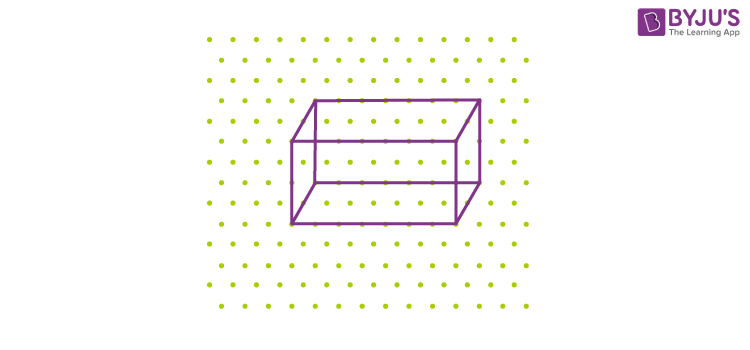5. According to the convention redraw the hidden edges as dotted lines as shown: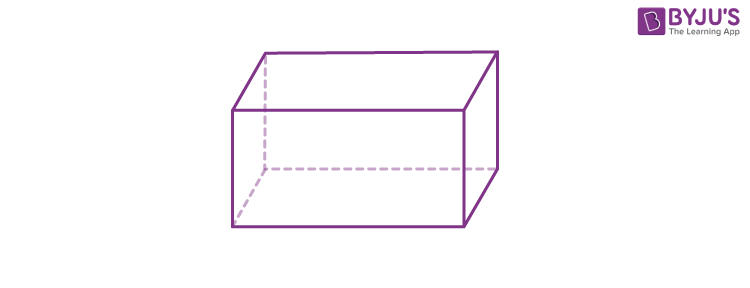This isometric sketch representing a cuboid of dimension 8 × 3 ×3. Thus, using an isometric dot sheet, we can draw three-dimensional shapes of exact measurements or dimensions without any ambiguity.

Thus, isometric sketches of different shapes can be drawn easily.